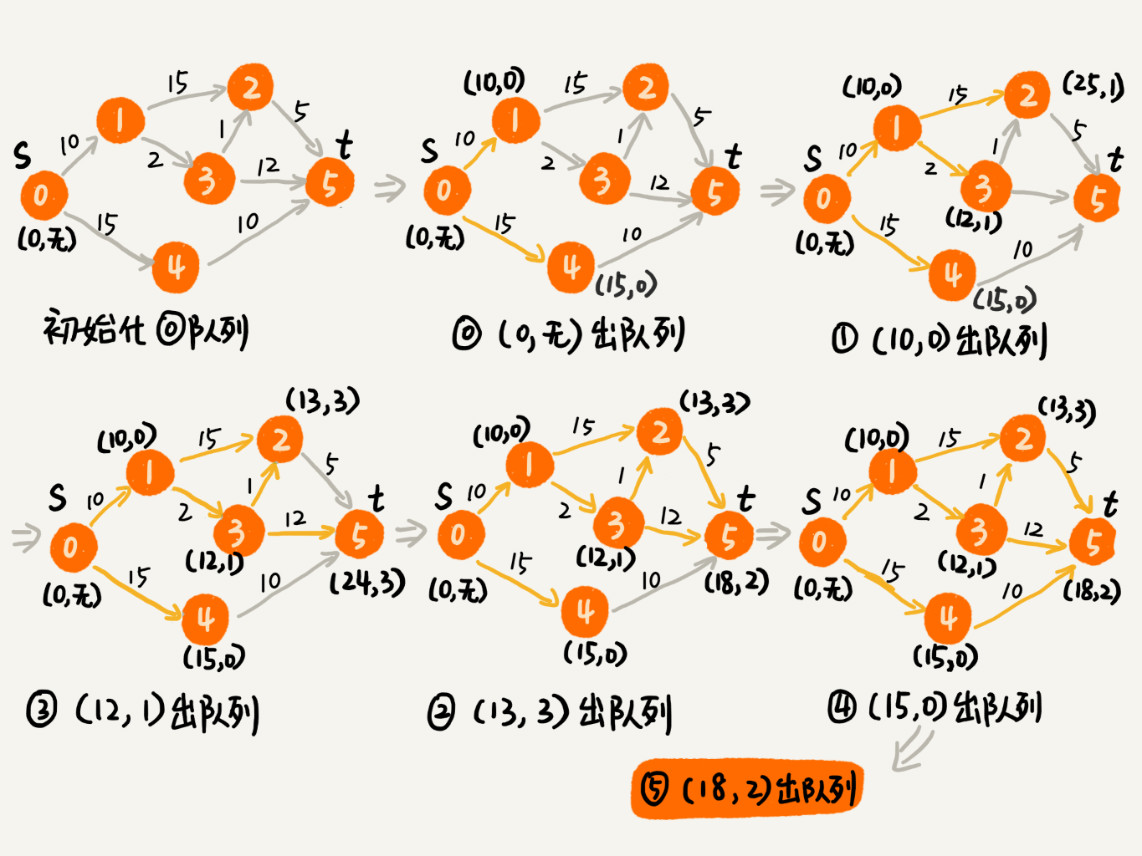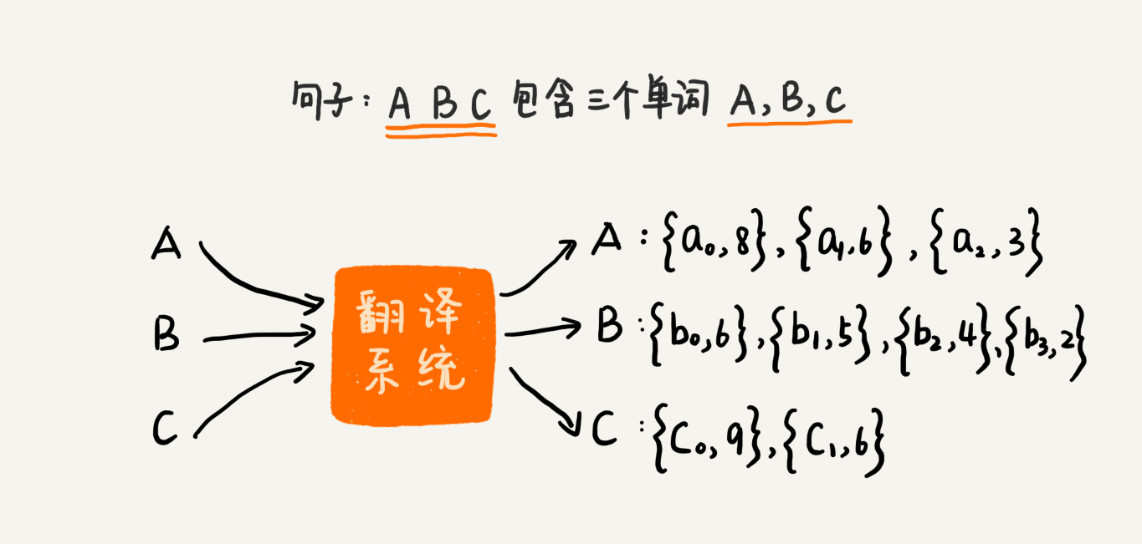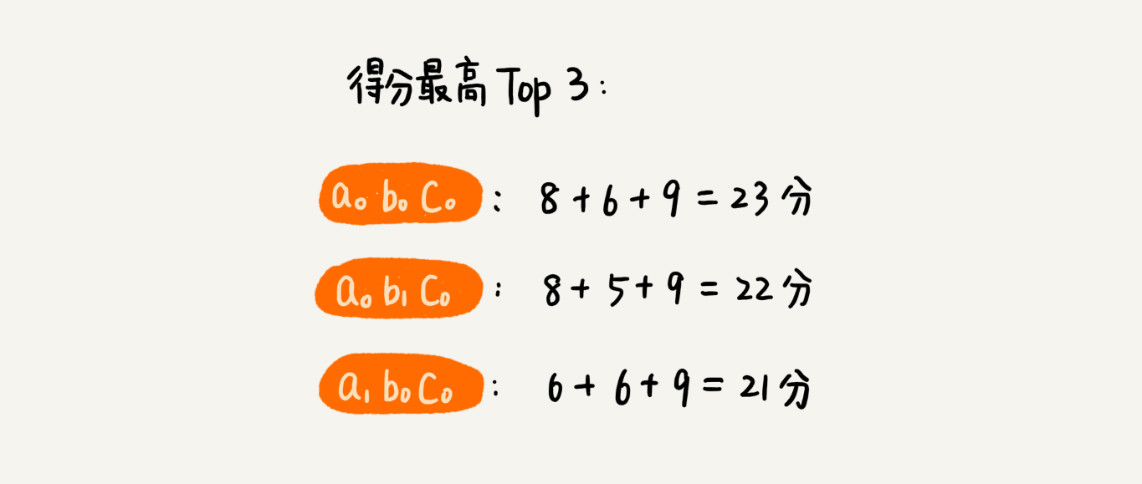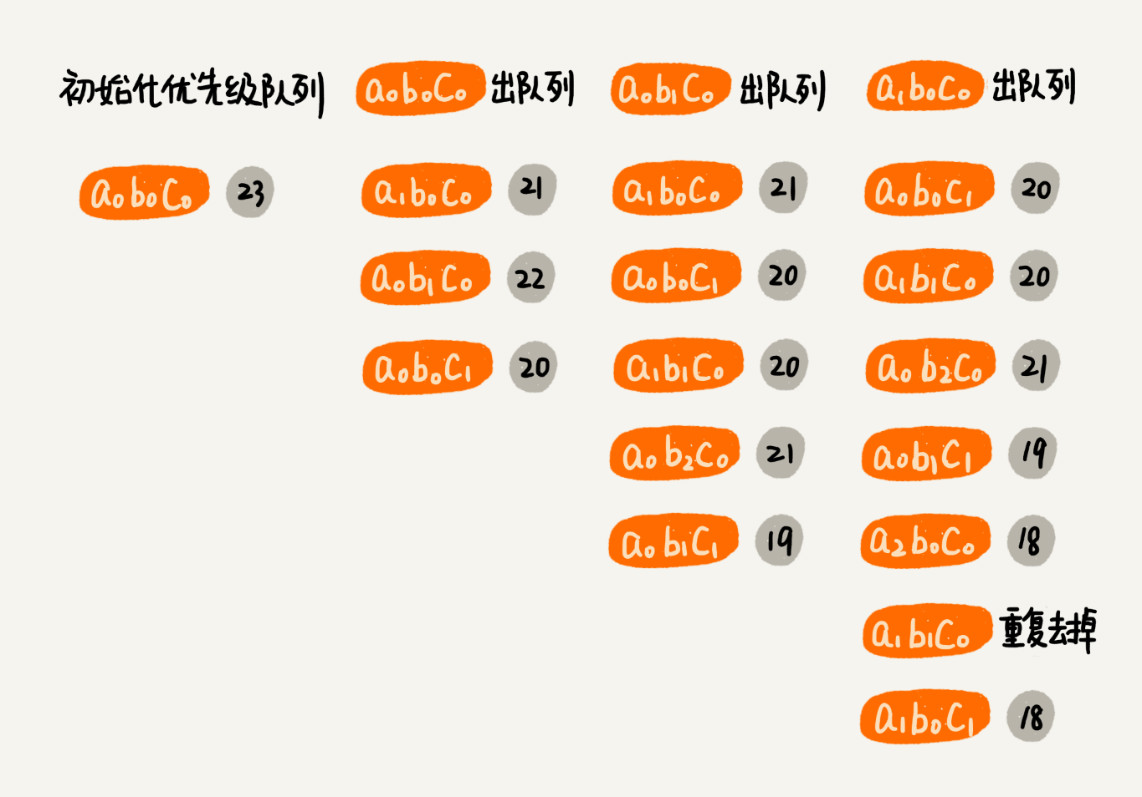`Google` 地图、百度地图、高德地图这样的地图软件，你应该经常使用吧？如果想从家开车到公司，你只需要输入起始、结束地址，地图就会给你规划一条最优出行路线。这里的最优，有很多种定义，比如最短路线、最少用时路线、最少红绿灯路线等等。作为一名软件开发工程师，你是否思考过，地图软件的最优路线是如何计算出来的吗？底层依赖了什么算法呢？

## 算法解析

``````public class Graph { // 有向有权图的邻接表表示
private int v; // 顶点个数

public Graph(int v) {
this.v = v;
for (int i = 0; i < v; ++i) {
}
}

public void addEdge(int s, int t, int w) { // 添加一条边
}

private class Edge {
public int sid; // 边的起始顶点编号
public int tid; // 边的终止顶点编号
public int w; // 权重
public Edge(int sid, int tid, int w) {
this.sid = sid;
this.tid = tid;
this.w = w;
}
}
// 下面这个类是为了 dijkstra 实现用的
private class Vertex {
public int id; // 顶点编号 ID
public int dist; // 从起始顶点到这个顶点的距离
public Vertex(int id, int dist) {
this.id = id;
this.dist = dist;
}
}
}``````

``````// 因为 Java 提供的优先级队列，没有暴露更新数据的接口，所以我们需要重新实现一个
private class PriorityQueue { // 根据 vertex.dist 构建小顶堆
private Vertex[] nodes;
private int count;
public PriorityQueue(int v) {
this.nodes = new Vertex[v+1];
this.count = v;
}
public Vertex poll() { // TODO: 留给读者实现... }
public void add(Vertex vertex) { // TODO: 留给读者实现...}
// 更新结点的值，并且从下往上堆化，重新符合堆的定义。时间复杂度 O(logn)。
public void update(Vertex vertex) { // TODO: 留给读者实现...}
public boolean isEmpty() { // TODO: 留给读者实现...}
}

public void dijkstra(int s, int t) { // 从顶点 s 到顶点 t 的最短路径
int[] predecessor = new int[this.v]; // 用来还原最短路径
Vertex[] vertexes = new Vertex[this.v];
for (int i = 0; i < this.v; ++i) {
vertexes[i] = new Vertex(i, Integer.MAX_VALUE);
}
PriorityQueue queue = new PriorityQueue(this.v);// 小顶堆
boolean[] inqueue = new boolean[this.v]; // 标记是否进入过队列
vertexes[s].dist = 0;
inqueue[s] = true;
while (!queue.isEmpty()) {
Vertex minVertex= queue.poll(); // 取堆顶元素并删除
if (minVertex.id == t) break; // 最短路径产生了
for (int i = 0; i < adj[minVertex.id].size(); ++i) {
Edge e = adj[minVertex.id].get(i); // 取出一条 minVetex 相连的边
Vertex nextVertex = vertexes[e.tid]; // minVertex-->nextVertex
if (minVertex.dist + e.w < nextVertex.dist) { // 更新 next 的 dist
nextVertex.dist = minVertex.dist + e.w;
predecessor[nextVertex.id] = minVertex.id;
if (inqueue[nextVertex.id] == true) {
queue.update(nextVertex); // 更新队列中的 dist 值
} else {
inqueue[nextVertex.id] = true;
}
}
}
}
// 输出最短路径
System.out.print(s);
print(s, t, predecessor);
}

private void print(int s, int t, int[] predecessor) {
if (s == t) return;
print(s, predecessor[t], predecessor);
System.out.print("->" + t);
}``````

`vertexes` 数组，记录从起始顶点到每个顶点的距离（`dist`）。起初，把所有顶点的 `dist` 都初始化为无穷大（也就是代码中的 `Integer.MAX_VALUE`）。把起始顶点的 `dist` 值初始化为 `0`，然后将其放到优先级队列中。

`predecessor` 数组的作用是为了还原最短路径，它记录每个顶点的前驱顶点。最后，通过递归的方式，将这个路径打印出来。打印路径的 `print` 递归代码就不详细讲了，这个跟在图的搜索中讲的打印路径方法一样。如果不理解的话，你可以回过头去看下那一篇。

`inqueue` 数组是为了避免将一个顶点多次添加到优先级队列中。更新了某个顶点的 `dist` 值之后，如果这个顶点已经在优先级队列中了，就不要再将它重复添加进去了。`for` 循环内部的代码涉及从优先级队列取数据、往优先级队列中添加数据、更新优先级队列中的数据，这样三个主要的操作。优先级队列是用堆来实现的，堆中的这几个操作，时间复杂度都是 \$O(logV)\$（堆中的元素个数不会超过顶点的个数 `V`）。

## 总结引申`k` 次出入队列，队列中的总数据不会超过 `k*n`，也就是说，出队、入队操作的时间复杂度是 \$O(log(k*n))\$。所以，总的时间复杂度就是 \$O(k * n * log(k * n))\$，比之前的指数级时间复杂度降低了很多。

## 思考

• 在计算最短时间的出行路线中，如何获得通过某条路的时间呢？

• 上面讲的出行路线问题，假设的是开车出行，那如果是公交出行呢？如果混合地铁、公交、步行，又该如何规划路线呢？欢迎留言和我分享。